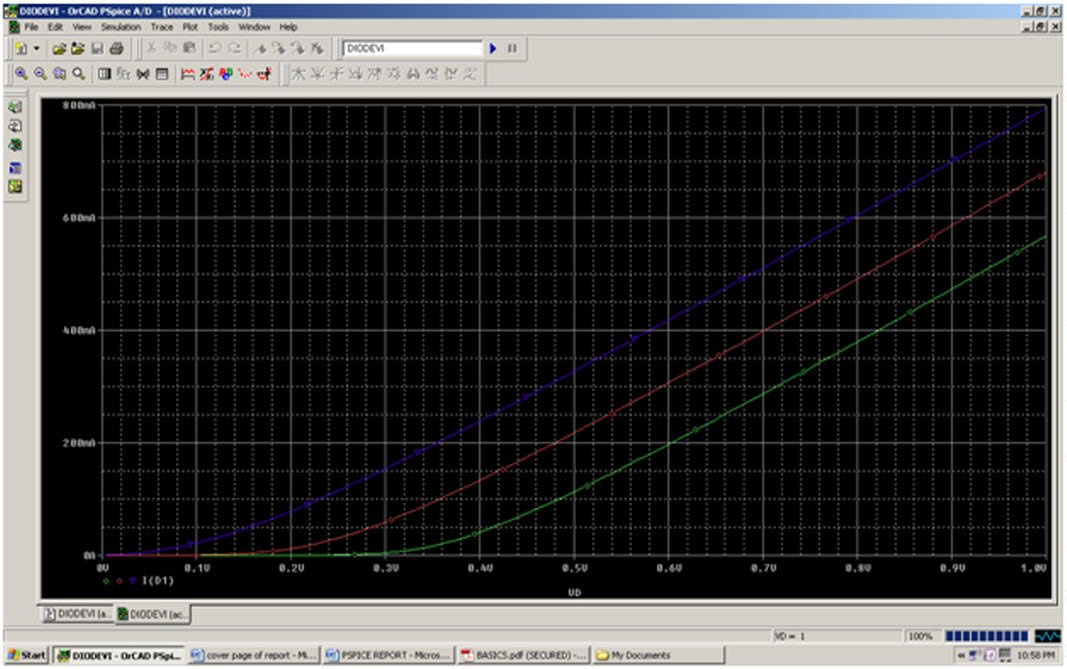# PSpice Textual Description

8. Simulate and study of DIODE characteristic in terms of temperature dependence.
*STUDY OF DIODE CHARECTERISTIC IN TERMS OF TEMP.

VD 1 0 DC 1V

D1 1 2 D1N914

VX 2 0 DC 0V

.MODEL D1N914 D(IS=3.93E-9 RS=1 BV=100V IBV=5E-6 CJO=1.7PF TT=2NS)

.TEMP 50 100 150

.DC VD 0V 1V 0.01V

.PLOT DC I(VX)

.PROBE

.END

GRAPHICAL  ANALYZER:

Special note:  In the graph Blue one for 50*c, Red one for 100*c and Green one for 150*cCONCLUSION:   From above characteristic we can conclude that DIODE drop decreases with the temperature  i.e. the DIODE current increases with the TEMPERATURE.

9. Study and simulation of CLIPPER CIRCUIT.

PSPICE CODING:

R1 1 2 .22K

D1 0 2 D1N3940

VAC 1 0 SIN(0V 5V 30KHZ)

.MODEL D1N3940 D(IS=4E-10 RS=.105 N=1.48 TT=8E-7 CJO=1.95E-11 VJ=0.4 M=.38 EG=1.36 XTI=-8 KF=0 AF=1 FC=.9 BV=600 IBV=1E-4)

.PROBE

.TRAN 0US 100US

.END

GRAPHICAL ANALYZER:CONCLUSION:  From above graph we can say that clipper circuit clip the negative part of the input signal(voltage) which is lie between -.8v to -5v so circuit is negative clipper circuit.

Posted in OrCAD PSpice and tagged , , .